# Transversal

In this chapter, you will learn about transversals, transversal lines and angles, and transversal examples to strengthen your concepts of transversals.

You check out the interactive examples to know more about the lesson and try your hand at solving a few interesting practice questions at the end of the page.

Before getting started, let's hear Jack's story.

Jack was driving from New Delhi to Agra, while Jill was driving on the same road from Agra to New Delhi.

Both roads on the highway that go to and fro are parallel.

Now, imagine these two roads are two parallel lines. Is it possible to calculate the distance between these two lines?

In fact, you can. Parallel lines are cut by a transversal. There are plenty of math transversal examples that we will see in this chapter, but before we get there, let us first understand what transversals are.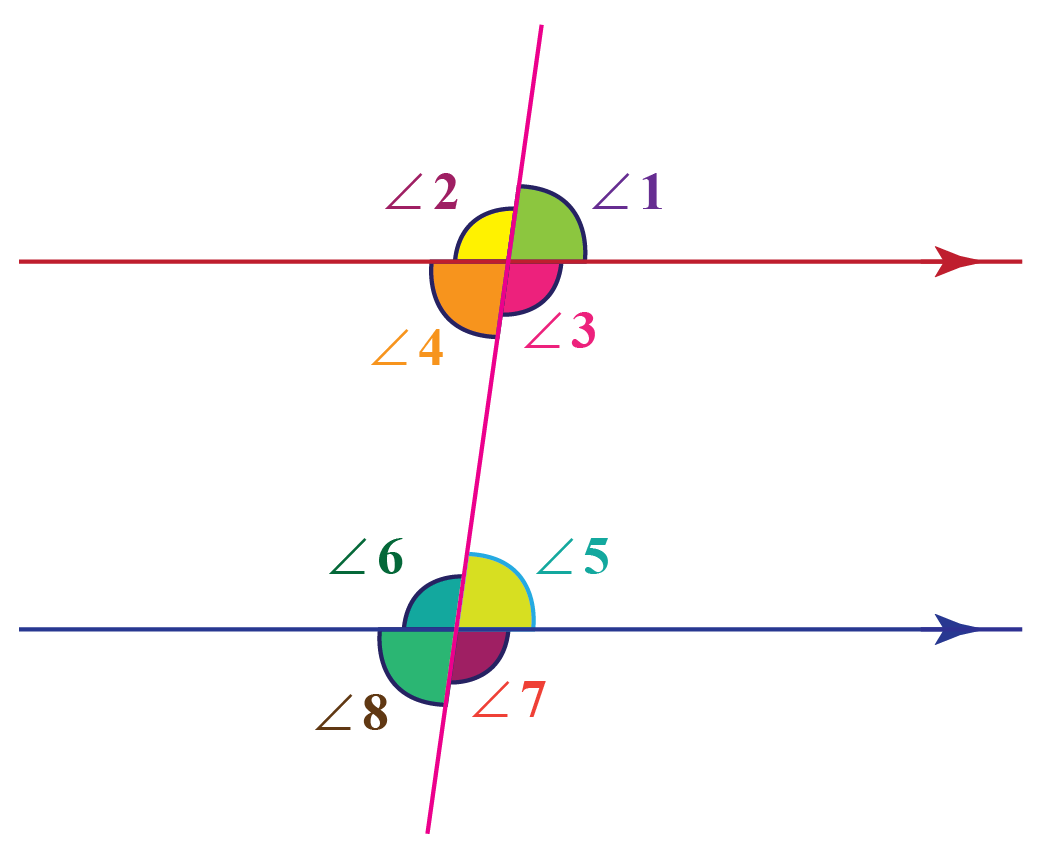Well, transversal is one of the main connecting links between any two lines (or parallel lines). In this article, we will learn about such transversals and how they help us in finding different properties of the two lines.

## Lesson Plan

 1 What are Transversals and Transverse Lines? 2 Solved Examples on Transversal 3 Interactive Questions on Transversal

## What are Transversals and Transverse Lines?

For two or more lines, a transversal is any line that intersects two lines at distinct points.

In the following figure, L1 and L2 are two lines that are cut by a transversal L. Here the line L is known as a transversal line. In the parallel and transversal line universe, the transversal line basically connects both parallel lines.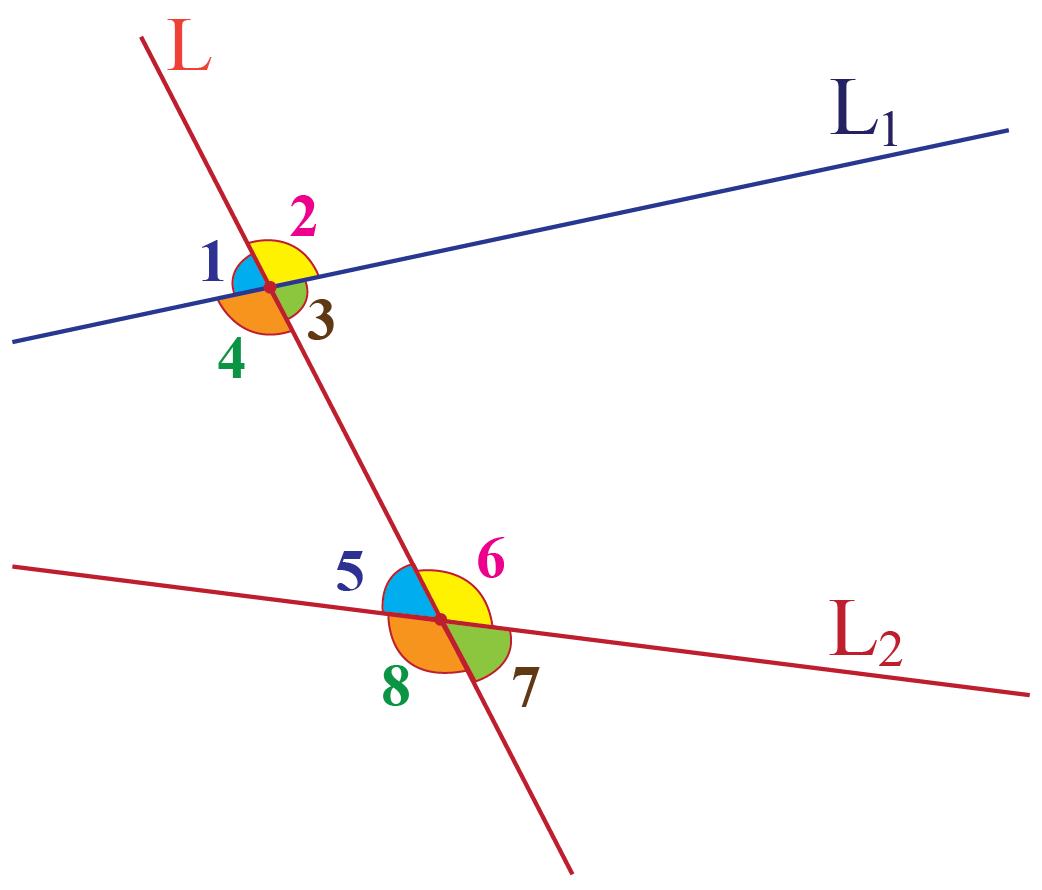From the diagram, we can say that 'L is a transversal that cuts the lines L1 and L2, and thus line L is the transversal line.'

## Construct a Transversal with Parallel Lines

The construction of a transversal is easy. First, we create two parallel lines.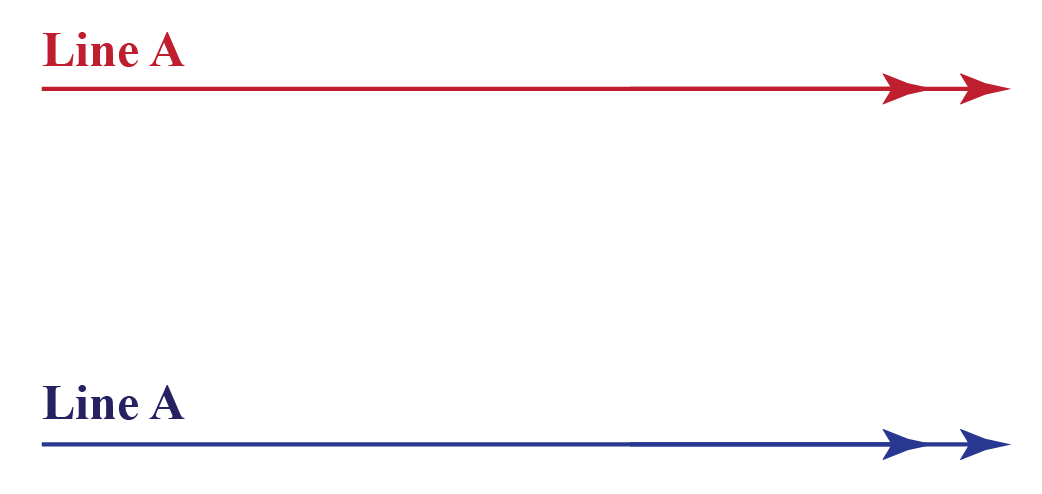Now, we construct the angle (at which we want to create the transversal) on the first line (say $$x^o$$) as shown.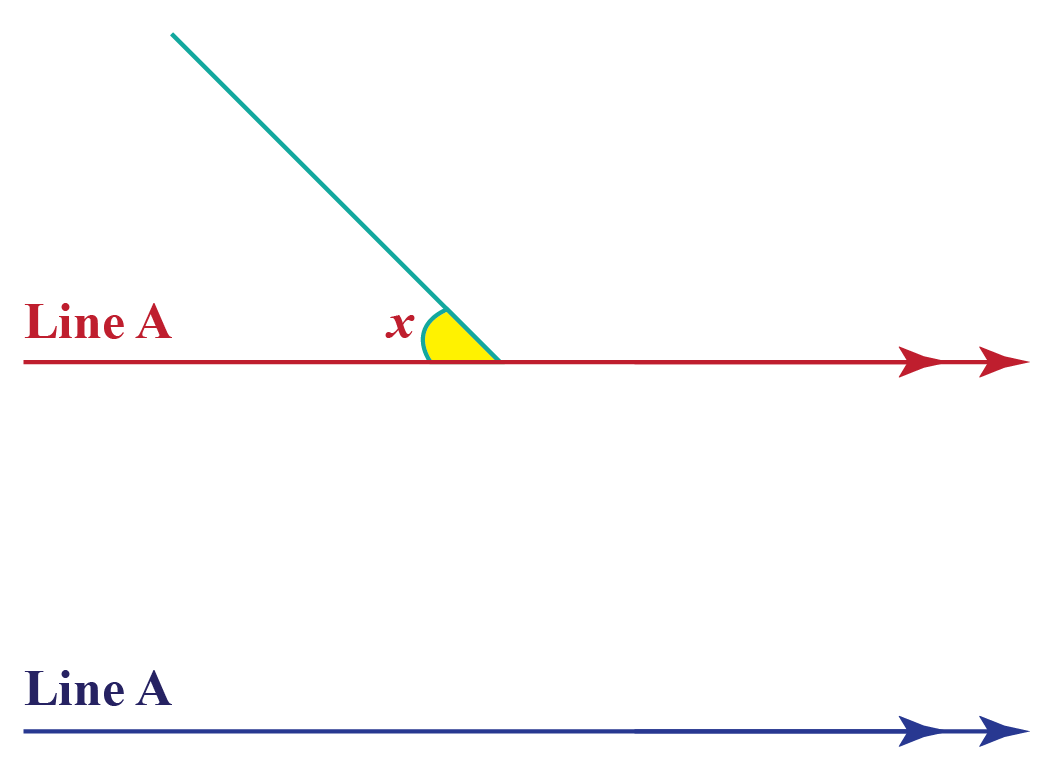Further, we extend this constructed angle up to the point it covers both the parallel lines as done here.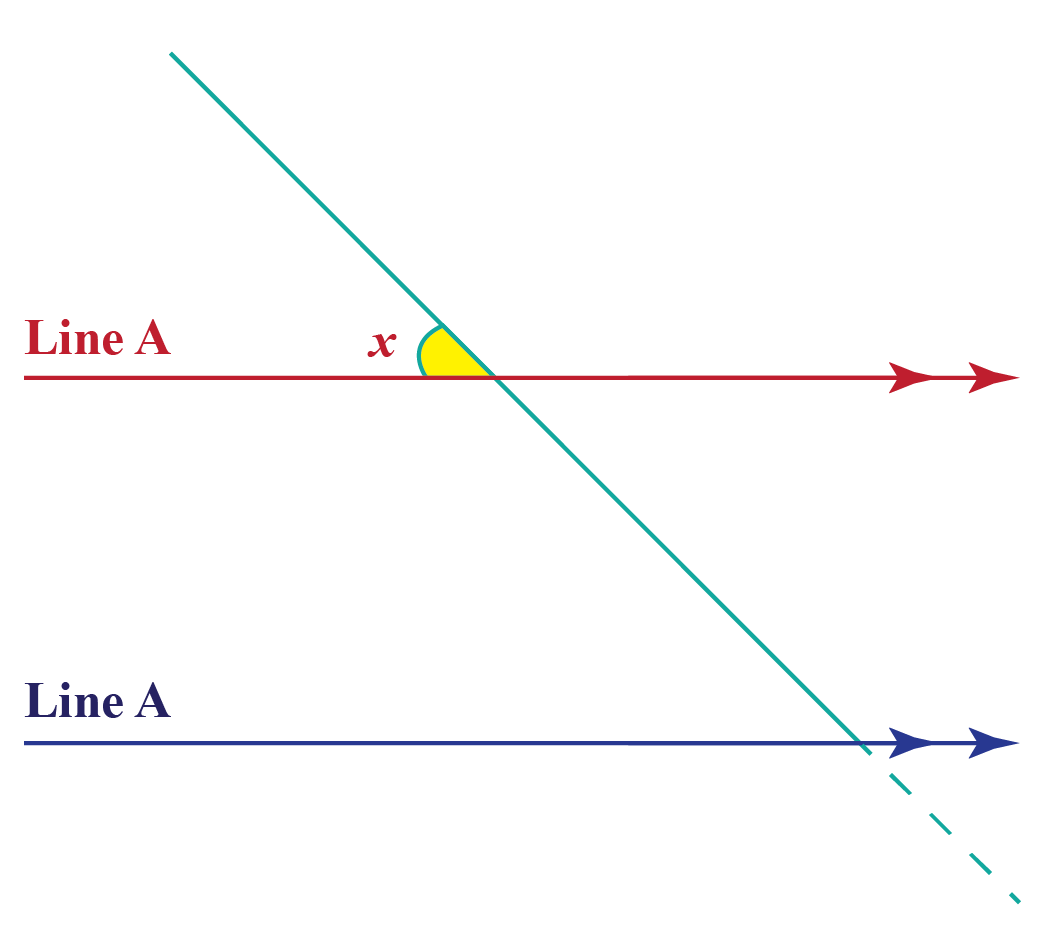So now here is what we get: Our transversal on the two parallel lines at the desired angle ($$x^o$$).

## What Are Transversal Angles?

When two parallel lines are cut by a transversal, a number of angles are formed by these two intersections.From the given diagram, if we try to organize the angles on the basis of the relative positions they occupy, we get the following categories of angles :

### Corresponding Angles

The following pairs of angles are corresponding angles:

• $$\angle 1$$ and $$\angle 5$$
• $$\angle 2$$ and $$\angle 6$$
• $$\angle 3$$ and $$\angle 7$$
• $$\angle4$$ and $$\angle 8$$

### Alternate Interior Angles

The following pairs of angles are alternate interior angles:

• $$\angle 3$$ and $$\angle 6$$
• $$\angle4$$ and $$\angle 5$$

### Alternate Exterior Angles

The following pairs of angles are alternate exterior angles:

• $$\angle 1$$ and $$\angle 8$$
• $$\angle 2$$ and $$\angle 7$$

### Co-interior Angles

The following pairs of angles are co-interior angles:

• $$\angle 3$$ and $$\angle 5$$
• $$\angle4$$ and $$\angle 6$$

## Interactive Questions

Here are a few activities for you to practice. Select/Type your answer and click the 'Check Answer' button to see the result.

## Let's Summarize

We hope you enjoyed learning about Transversal with the solved examples and practice questions. Now you will be able to easily solve problems on transversal examples, parallel lines cut by a transversal, transversal lines and angles, transversal and parallel lines.

## About Cuemath

At Cuemath, our team of math experts is dedicated to making learning fun for our favorite readers, the students!

Through an interactive and engaging learning-teaching-learning approach, the teachers explore all angles of a topic.

Be it worksheets, online classes, doubt sessions, or any other form of relation, it’s the logical thinking and smart learning approach that we, at Cuemath, believe in.

## 1. Can transversal lines be straight?

Yes, in fact, transversal lines have to be straight lines that stretch throughout the given parallel lines.

## 2. How many angles are formed by the transversal?

There are a number of angles formed by the transversal. These are
a) Corresponding Angles
b) Alternate Interior Angles
c) Alternate Exterior Angles
d) Co-interior Angles

## 3. Do transversal lines have to be parallel?

No. Parallel and transversal lines are different. Transversal lines can be at any angle to the given parallel lines. Thus, any 2 transversals need not be parallel.

More Important Topics
Numbers
Algebra
Geometry
Measurement
Money
Data
Trigonometry
Calculus